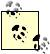# Estimating Rate of Return

Problem

You're evaluating a series of payments and cash flows and want to compute the rate of return on your investment.

Solution

Use the IRR function to compute the internal rate of return .

Discussion

Let's reconsider the example cash flow options discussed in Recipe 14.6. In addition to looking at net present values, you also want to compute the rate of return on each investment. IRR will do that for you. The syntax for IRR is =IRR(values, guess), where values is a cell range containing the investment and cash flow value and guess is your initial guess at the rate of return.IRR uses an iterative solution approach; your initial guess is used to help it converge on a solution.

To compute the rates of return for the two projects, project A and project B, enter these two formulas: =IRR(C8:C12) and =IRR(D8:D12). The first formula computes the rate of return for project A before discounting, and the second formula computes the rate of return for project B before discounting. These formulas return 50% and 43%, respectively, indicating that project A is the better option.

The formulas =IRR(E8:E12) and =IRR(F8:F12) compute the rates of return for projects A and B after discounting their cash flows. The resulting rates of return are 43% and 36% for project A and project B, respectively. These results again indicate that project A is the better alternative.Excel Scientific and Engineering Cookbook (Cookbooks (OReilly))
ISBN: 0596008791
EAN: 2147483647
Year: N/A
Pages: 206
Authors: David M Bourg